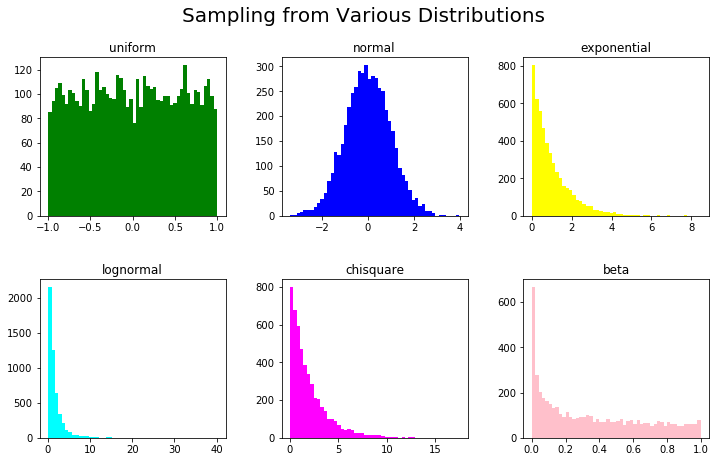# Generating Synthetic Data with Numpy and Scikit-LearnIn this tutorial, we'll discuss the details of generating different synthetic datasets using Numpy and Scikit-learn libraries. We'll see how different samples can be generated from various distributions with known parameters.

#### Introduction

In this tutorial, we'll discuss the details of generating different synthetic datasets using Numpy and Scikit-learn libraries. We'll see how different samples can be generated from various distributions with known parameters.

We'll also discuss generating datasets for different purposes, such as regression, classification, and clustering. At the end we'll see how we can generate a dataset that mimics the distribution of an existing dataset.

##### The Need for Synthetic Data

In data science, synthetic data plays a very important role. It allows us to test a new algorithm under controlled conditions. In other words, we can generate data that tests a very specific property or behavior of our algorithm.

For example, we can test its performance on balanced vs. imbalanced datasets, or we can evaluate its performance under different noise levels. By doing this, we can establish a baseline of our algorithm's performance under various scenarios.

There are many other instances, where synthetic data may be needed. For example, real data may be hard or expensive to acquire, or it may have too few data-points. Another reason is privacy, where real data cannot be revealed to others.

#### Setting Up

Before we write code for synthetic data generation, let's import the required libraries:

``````import numpy as np

## Needed for plotting
import matplotlib.colors
import matplotlib.pyplot as plt
from mpl_toolkits.mplot3d import Axes3D

## Needed for generating classification, regression and clustering datasets
import sklearn.datasets as dt

## Needed for generating data from an existing dataset
from sklearn.neighbors import KernelDensity
from sklearn.model_selection import GridSearchCV``````

Then, we'll have some useful variables in the beginning:

``````## Define the seed so that results can be reproduced
seed = 11
rand_state = 11

## Define the color maps for plots
color_map = plt.cm.get_cmap('RdYlBu')
color_map_discrete = matplotlib.colors.LinearSegmentedColormap.from_list("", ["red","cyan","magenta","blue"])``````

## Scikit-Learn Course - Machine Learning in Python Tutorial

Learn about machine learning using scikit-learn. Scikit-learn is a free software machine learning library for the Python programming language: Getting Started with Machine Learning, Taking a look at some machine learning algorithms, Artificial Intelligence and the science behind It

## Scikit-Learn (Python): 6 Useful Tricks for Data Scientists

Scikit-Learn (Python): 6 Useful Tricks for Data Scientists. Tricks to improve your machine learning models in Python with scikit-learn (sklearn).

## Everything You Need to Know About Scikit-Learn Python library

Everything You Need to Know About Scikit-Learn Python library. Take a look at the Scikit-Python library, including its implementation, training a model, and some additional tips.

## Learning Python: The Prompt, Then Read Template

The most Python programs will consist of three steps — getting input into the program, processing the input in some way, and outputting the results of the processing. I’m going to focus on one part of that step — getting input into a program — by prompting the user to enter some data and then reading the data into the program. This is a mostly straightforward process except for some data conversions that have to occur when you are inputting numbers.

## Python Tricks Every Developer Should Know

In this tutorial, you’re going to learn a variety of Python tricks that you can use to write your Python code in a more readable and efficient way like a pro.